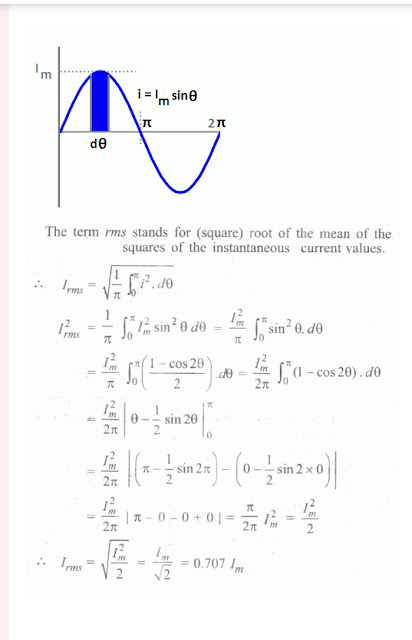RMS Value of AC | Average Value of AC | Difference Between RMS and Average Value - Electrical Diary -->

# RMS Value of AC | Average Value of AC | Difference Between RMS and Average Value

## What is the RMS Value of AC?|Definiton of RMS

The alternating voltage or current wave shape completes a certain number of cycles in one second, each cycle consisting of the identical positive and negative half cycle.

The value of the quantity in such a wave shape changes from instant to instant.it becomes maximum at a certain instant only. In specifying such a varying quantity Either voltage or current, it's maximum or peak value rarely used.

The root means square (RMS) value of the alternating current or voltage is more extensively used in practice to express the quantity. During measurement MI ammeter and Voltmeter register this quantity only for all types of alternating current voltage waves.

Normally the current is measured by the amount of work it will do or the amount of heat it will produce. Thus the RMS or effective value of alternating current is defined as that value of direct current which will do the same amount of work at the same time or would produce the same heating effect as when the alternating current is applied.

Usually, the value of alternating current and voltage is taken as RMS or effective value unless otherwise specified. while representing the RMS value of current and voltage simple symbol V and I are used.

### Derivation of Root mean Value of Sine Wave | RMS Value of AC Currentimage credit electricaleasy.com

From the above-given Derivation, it is clear that the RMS Value of the Alternating current is 0.707 times the maximum value of the AC current.

### RMS Value of Voltage | RMS Voltage

Same as that of Alternating Current, RMS Voltage is also calculated for Alternating Voltage. RMS Voltage is 0.707 times the Maximum Value of alternating voltage same as that of RMS Value of Alternating Current and is given as

VRMS=0.707 Vmax

### What is the Average Value of Alternating Current and Voltage?

The average value of the current or voltage of an alternating wave shape is the alternating mean of the orginates at equal intervals over a half cycles of that wave. if we try to find out the Average value of either voltage or current of an alternating quantity for a complete period of time will be zero if the wave shape of alternating quantity is symmetrical.

In such a condition the current will be positive for half period of time and for the next half period of time it will be negative. This is the reason why Alternating Current or Voltage is not used for charging the battery.

but if we rectify the alternating current then we shall get fluctuated but unidirectional current and then the Average value of such a current have a definite magnitude over a complete period of time and this rectified current can be used for electro-chemical work or other purposes.

The average value  AC Current of a sine wave is defined as 0.637 times the maximum value of the sine wave and mathematically it can be represented as

Iavg=0.637Imax

and this relation is the same in the case of Alternating Voltage (Average Value of Voltage) and It can be also mathematically represented as

Vavg=0.637Vmax

### Form Factor | Definition of Form Factor

the form factor is defined as the ratio of RMS value to the Average Value of a sine wave of Either Voltage or Current. the form factor of A sine wave is calculated as
Form Factor = 0.707Imax /0.637Imax
The form factor for Sine wave =1.11